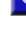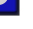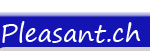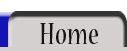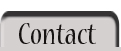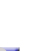All Operators > Operator < Operator <= OperatorOPERATOR:   >= Implemented in version 1.0   >=   The >= operator determines if the first expression is greater-than-or-equal the second expression.   Code: <% 123 >= 456 %> <% "a" >= "A" %>   Output: False True